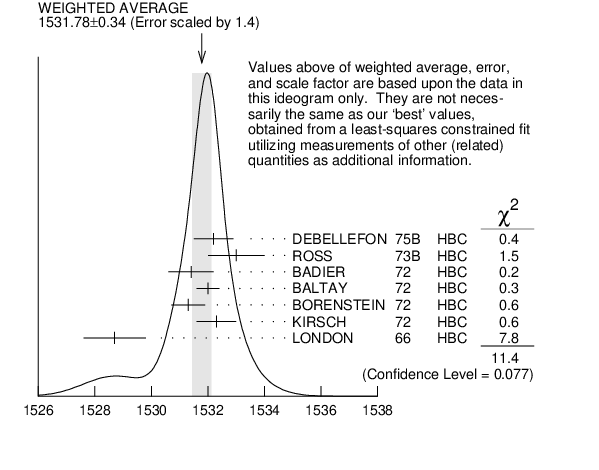# ${{\boldsymbol \Xi}{(1530)}^{0}}$ MASS INSPIRE search

VALUE (MeV) EVTS DOCUMENT ID TECN  COMMENT
$\bf{ 1531.80 \pm0.32}$ OUR FIT  Error includes scale factor of 1.3.
$\bf{ 1531.78 \pm0.34}$ OUR AVERAGE  Error includes scale factor of 1.4.
$1532.2$ $\pm0.7$
 1975 B
HBC ${{\mathit K}^{-}}$ ${{\mathit p}}$ $\rightarrow$ ${{\mathit \Xi}^{-}}{{\overline{\mathit K}}}{{\mathit \pi}}$
$1533$ $\pm1$
 1973 B
HBC ${{\mathit K}^{-}}$ ${{\mathit p}}$ $\rightarrow$ ${{\mathit \Xi}}{{\overline{\mathit K}}}{{\mathit \pi}}$( ${{\mathit \pi}}$)
$1531.4$ $\pm0.8$ 59
 1972
HBC ${{\mathit K}^{-}}{{\mathit p}}$ 3.95 ${\mathrm {GeV/}}\mathit c$
$1532.0$ $\pm0.4$ 1262
 1972
HBC ${{\mathit K}^{-}}{{\mathit p}}$ 1.75 ${\mathrm {GeV/}}\mathit c$
$1531.3$ $\pm0.6$ 324
 1972
HBC ${{\mathit K}^{-}}{{\mathit p}}$ 2.2 ${\mathrm {GeV/}}\mathit c$
$1532.3$ $\pm0.7$ 286
 1972
HBC ${{\mathit K}^{-}}{{\mathit p}}$ 2.87 ${\mathrm {GeV/}}\mathit c$
$1528.7$ $\pm1.1$ 76
 1966
HBC ${{\mathit K}^{-}}{{\mathit p}}$ 2.24 ${\mathrm {GeV/}}\mathit c$
• • • We do not use the following data for averages, fits, limits, etc. • • •
$1532.1$ $\pm0.4$ 1244
 1985 B
LASS ${{\mathit K}^{-}}{{\mathit p}}$ 11 ${\mathrm {GeV/}}\mathit c$
$1532.1$ $\pm0.6$ 2700 1
 1981 B
HBC ${{\mathit K}^{-}}{{\mathit p}}$ 8.25 ${\mathrm {GeV/}}\mathit c$
$1530$ $\pm1$ 450
 1981
SPEC SPS hyperon beam
$1527$ $\pm6$ 80
 1979
HBC ${{\mathit K}^{-}}{{\mathit p}}$ 10 ${\mathrm {GeV/}}\mathit c$
$1535$ $\pm4$ 100
 1979
HBC ${{\mathit K}^{-}}{{\mathit p}}$ 16 ${\mathrm {GeV/}}\mathit c$
$1533.6$ $\pm1.4$ 97
 1974
HBC Quasi-2-body $\sigma{}$
1  BAUBILLIER 1981B is a fit to the inclusive spectrum. The resolution (5 MeV) is not unfolded.${{\mathit \Xi}{(1530)}^{0}}$ mass (MeV)
References:
 ASTON 1985B
PR D32 2270 Inclusive Production of Multistrange Hyperons from 11 ${\mathrm {GeV/}}\mathit c$ ${{\mathit K}^{-}}{{\mathit p}}$ Interactions
 BAUBILLIER 1981B
NP B192 1 A Study of Inclusive ${{\mathit \Xi}^{-}}$, ${{\mathit \Xi}{(1530)}}$ and ${{\mathit \Omega}^{-}}$ Production in ${{\mathit K}^{-}}{{\mathit p}}$ Interactions at 8.25 ${\mathrm {GeV/}}\mathit c$
 BIAGI 1981
ZPHY C9 305 Production of Hyperons and Hyperon Resonances in ${{\mathit \Xi}^{-}}{{\mathit N}}$ Interactions at 102 and 135 ${\mathrm {GeV/}}\mathit c$
 SIXEL 1979
NP B159 125 Inclusive Production of ${{\mathit \Xi}^{-}}$ and ${{\mathit \Xi}^{*}{(1530)}}$ in ${{\mathit K}^{-}}{{\mathit p}}$ Interactions at 10 and 16 ${\mathrm {GeV/}}\mathit c$
 DEBELLEFON 1975B
NC 28A 289 Reactions ${{\mathit K}^{-}}$ ${{\mathit p}}$ $\rightarrow$ ${{\mathit \Xi}^{-}}{{\mathit K}^{0}}{{\mathit \pi}^{+}}$ between 2210 and 2435 MeV $\mathit E_{{\mathrm {cm}}}$
 BERTHON 1974
NC 21A 146 Cross Sections for Quasi-two-body Reactions in ${{\mathit K}^{-}}{{\mathit p}}$ Interactions between 1263 and 1843 ${\mathrm {MeV}}/\mathit c$
 ROSS 1973B
Purdue Conf. 355 ${{\mathit \Xi}{(1530)}^{0}}$ Resonance in Reactions at 3.1 to 3.6 ${\mathrm {GeV/}}\mathit c$
NP B37 429 Search for ${{\mathit \Xi}^{*}}$ Resonances in ${{\mathit K}^{-}}{{\mathit p}}$ Interactions at 3.95 ${\mathrm {GeV/}}\mathit c$
PL 42B 129 Measurement of the ${{\mathit \Xi}^{*}{(1530)}}$ Mass, Width, and Mass Difference
PR D5 1559 Reaction ${{\mathit K}^{-}}$ ${{\mathit p}}$ $\rightarrow$ ${{\mathit \Xi}^{-}}{{\mathit K}^{0}}{{\mathit \pi}^{+}}$ at 2.18 ${\mathrm {GeV/}}\mathit c$
NP B40 349 The Mass and Width of the ${{\mathit \Xi}{(1530)}}$
PR 143 1034 ${{\mathit K}^{-}}{{\mathit p}}$ Interaction at 2.24 BeV